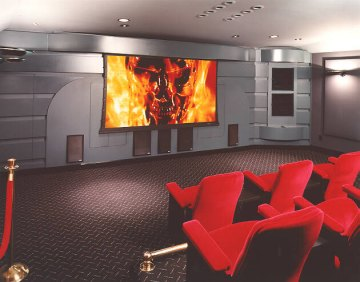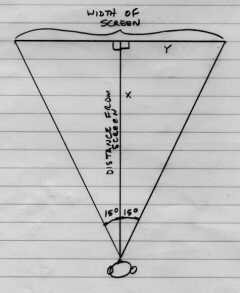Home Theater Screen Width and Viewing Distance Calculations

 I get lots of questions asking how far back my seats are from the screen, and how I established the distance. My research concentrated on where the ideal position would be in a regular theater. I remember that I found a discussion of this calculation in The Perfect Vision that suggested the distance be calculated using screen height since the width would change depending on the aspect ratio. As I recall the recommendation was 3.5 x the screen height. Since I wanted to test it out at a normal film theater, but could not easily determine the actual height of the installed screen, I converted the 3.5 x height calculation to a 30 degree horizontal angle from my point of view. I checked it out at a theater. Perfect. I decided that calculating the width using the max horizontal width, as opposed to the screen height is better for widescreen movies since it allows you to make the same calculation whether you have a variable aspect ratio device or not. Also, if you decide on a 1.78:1 aspect ratio screen, I feel the width should be still have the same 30 degree point of view angle, and this takes that into account. So, wrapping it up, I recommend you use the 30 degree horizontal guideline. Here is a sketch I made to show how this works, along with the appropriate calculations.I used a scientific calculator in my calculations, but I have included the necessary numbers so it is not required, unless you want to use another angle. Referring to the sketch above, X = distance from the screen to your seat. Y = 1/2 the width of the screen. If you know X; Y = Tangent of 15 degrees times X. Double Y for the screen width. This is what you use if you have a fixed distance between the screen and the seating position. If you know Y; X = Y divided by the tangent of 15 degrees. Use this if you have a desired screen size and want to know the optimum distance to your seats. Don't forget that Y is 1/2 your screen width. The tangent of 15 degrees is .2679 Back to Aspect Ratios Copyright 1996-2001, Philip Wise. All Rights Reserved.